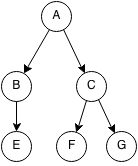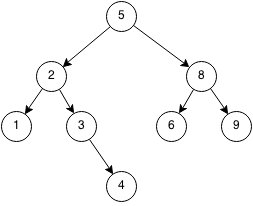## Introduction

A binary tree is a tree such that each node has at most 2 children.## Binary Search Tree

A binary search tree is a type of binary tree where all the nodes in a left subtree will be smaller than the node and all the nodes in a right subtree will be greater than the node. It has a recursive structure such that each subtree is also a binary search tree.